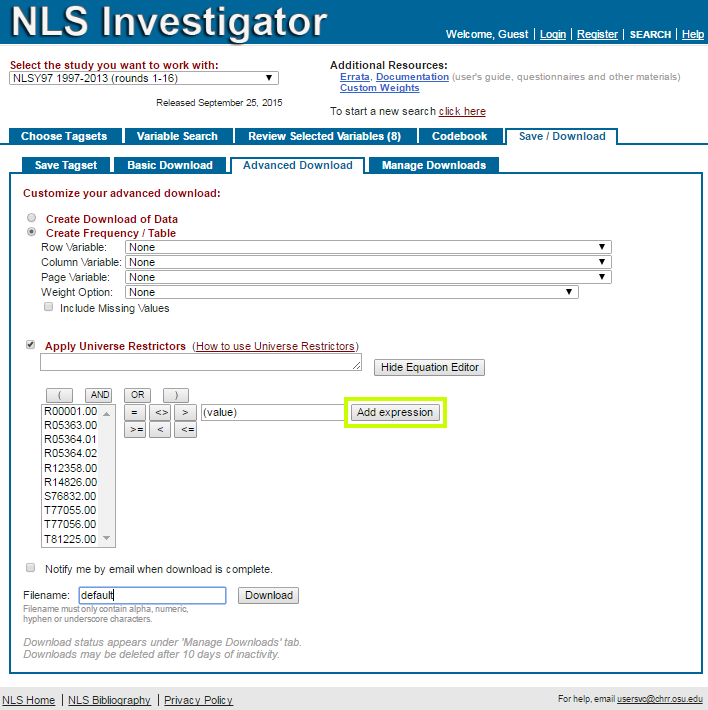# Apply Universe Restrictors

When using functions from the Advanced Download sub-tab, you can apply universe restrictors to download only a subset of the data set. For example, you might only want to look at women if you are researching pregnancy.

Check the square box to the left of the "Apply Universe Restrictors" menu bar to enter the desired restrictors. Either enter the restrictors directly in the box or click on "Show Equation Editor" to help create the restrictors. Note that you must click "Add expression" after using the equation editor to set up your restrictors.Important:

• Any variables you use within restrictors must be in your tagset
• If you want to use more than one operand ("AND" or "OR"), you must use parentheses:

For restrictors with two or more operands, write:

R0000100 = 1 OR (R0000200 = 1 OR R0000300 = 1)
(R0000100 = 1 AND R0000200 = 1) OR R0000300 = 1
(R0000100 = 1 AND R0000200 = 1) OR (R0000300 = 1 AND R0000400 = 1)